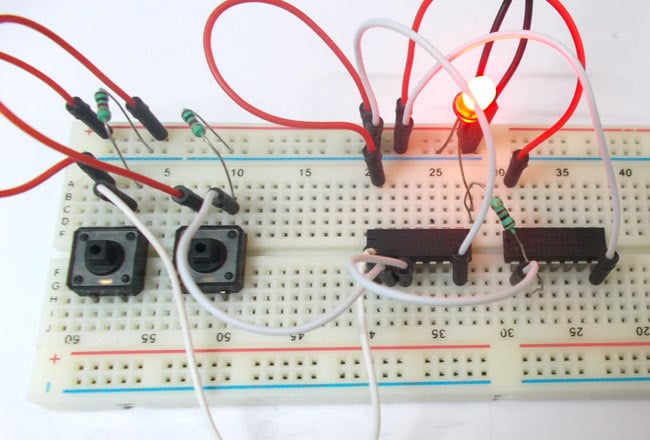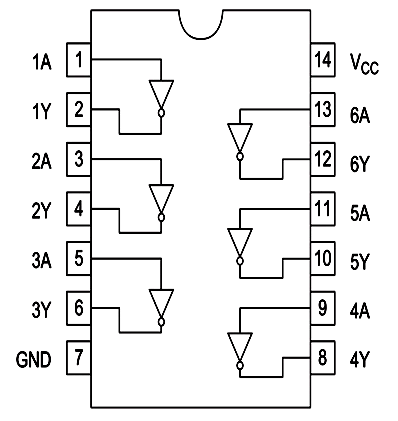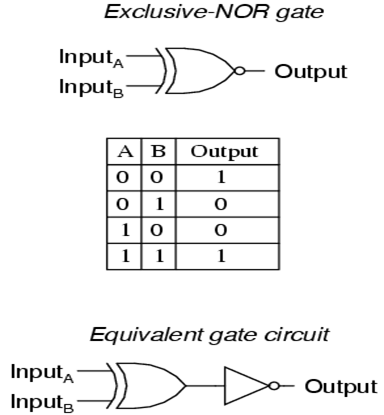# XNOR Gate

Published  September 13, 2015   0XNOR or EX-NOR gate is a digital logic gate, designed for arithmetic and logical operations. Here we are going to use 74LS86 chip for demonstration, this chip has 4 EX-OR gates in it. These four gate are connected internally as shown in below figure.These EX-OR gates are followed by NOT gates. These gates provides inverted output of EX-OR gate, so with both, we will have EX-NOR gate. Here we use 74LS04 HEX NOT gate chip. Pin diagram of 74LS04 IC is shown below.### Components

Power supply (5v), 1K resistor (2 pieces), 220Ω resistor, 74LS86 QUAD EX-OR GATE IC, 74LS04 HEX NOT gate chip,1 LEDS, buttons (2 pieces), 100nF capacitor (2 pieces) Connecting wires, breadboard.

### EXNOR Gate Circuit Diagram and Working Explanation

The truth table of EX-NOR gate is show in below figure.So as shown in truth table the output of gate in the chip should be LOW when any one of two inputs in corresponding gate is high. In EX-NOR gate the output will be HIGH if both inputs are either high or LOW.

In this EXNOR gate circuit we are going to pull down both input of a gate to ground through a 1KΩ resistor.  And then the inputs are connected to power through a button. So when the button is pressed the corresponding pin of gate goes high. So with two buttons we can realize the truth table of EX-NOR gate. When one of button is pressed one input of gate will be high and other will be low at this time the output should be LOW.

The capacitor is for neutralizing the bouncing effect of the button. In the absence of capacitor, the counter may count the events occurring wrongly.These pull down resistors are necessary as the chosen CHIP is a positive edge triggering one. If the resistors are ignored the circuit might generate unpredictable results.

The capacitors here are for neutralizing the bouncing effect of buttons. Although the capacitors here are not compulsory, putting them might smooth the working of EXNOR gate.

Video